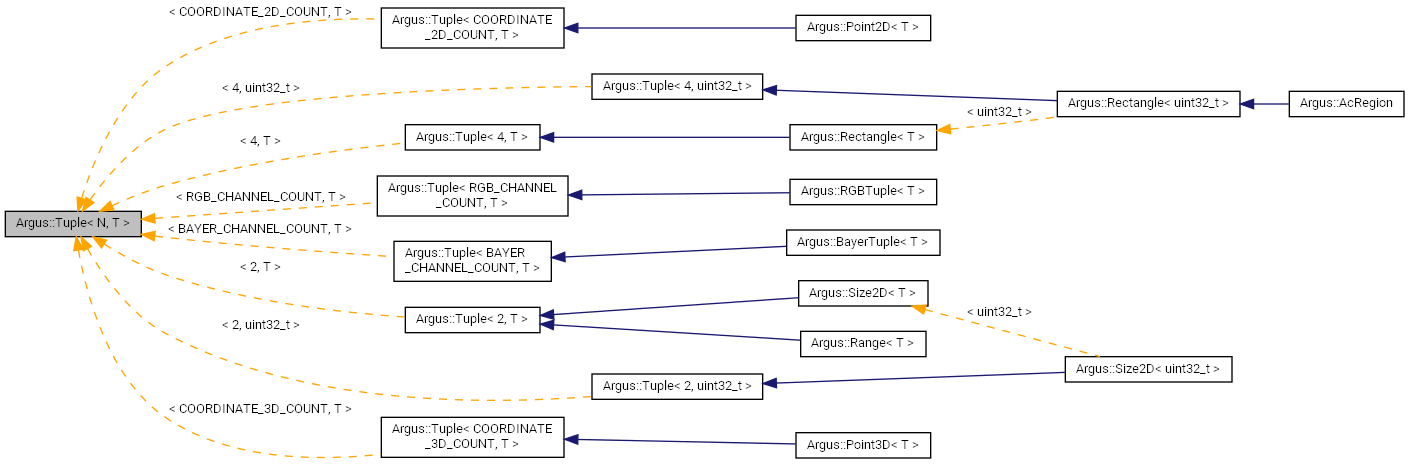## L4T Multimedia API Reference

#### 32.2 Release

Argus::Tuple< N, T > Class Template Reference

## Detailed Description

### template<unsigned int N, typename T> class Argus::Tuple< N, T >

Tuple template class.

This provides a finite ordered list of N elements having type T.

Definition at line 444 of file Types.h.

Inheritance diagram for Argus::Tuple< N, T >:[legend]
Collaboration diagram for Argus::Tuple< N, T >:[legend]

## Public Member Functions

Tuple ()

Tuple (T init)
Initialize every element of the tuple to a single value. More...

bool operator== (const Tuple< N, T > &rhs) const
Returns true when every element in the two tuples are identical. More...

bool operator!= (const Tuple< N, T > &rhs) const
Returns true if there are any differences between the two tuples. More...

Tuple< N, T > & operator+= (const Tuple< N, T > &rhs)
Adds every element of another tuple to the elements of this tuple. More...

Tuple< N, T > & operator-= (const Tuple< N, T > &rhs)
Subtracts every element of another tuple from the elements of this tuple. More...

Tuple< N, T > & operator*= (const T &rhs)
Multiplies every element in the tuple by a single value. More...

Tuple< N, T > & operator/= (const T &rhs)
Divides every element in the tuple by a single value. More...

const Tuple< N, T > operator+ (const Tuple< N, T > &rhs) const
Returns the result of adding another tuple to this tuple. More...

const Tuple< N, T > operator- (const Tuple< N, T > &rhs) const
Returns the result of subtracting another tuple from this tuple. More...

const Tuple< N, T > operator* (const T &rhs) const
Returns the result of multiplying this tuple by a single value. More...

const Tuple< N, T > operator/ (const T &rhs) const
Returns the result of dividing this tuple by a single value. More...

T & operator[] (unsigned int i)

const T & operator[] (unsigned int i) const

## Static Public Member Functions

static unsigned int tupleSize ()
Returns the number of elements in the tuple. More...

m_data [N]

## Constructor & Destructor Documentation

template<unsigned int N, typename T>
 Argus::Tuple< N, T >::Tuple ( )
inline

Definition at line 447 of file Types.h.

template<unsigned int N, typename T>
 Argus::Tuple< N, T >::Tuple ( T init )
inline

Initialize every element of the tuple to a single value.

Definition at line 450 of file Types.h.

## Member Function Documentation

template<unsigned int N, typename T>
 bool Argus::Tuple< N, T >::operator!= ( const Tuple< N, T > & rhs ) const
inline

Returns true if there are any differences between the two tuples.

Definition at line 463 of file Types.h.

template<unsigned int N, typename T>
 const Tuple Argus::Tuple< N, T >::operator* ( const T & rhs ) const
inline

Returns the result of multiplying this tuple by a single value.

Definition at line 513 of file Types.h.

template<unsigned int N, typename T>
 Tuple& Argus::Tuple< N, T >::operator*= ( const T & rhs )
inline

Multiplies every element in the tuple by a single value.

Definition at line 485 of file Types.h.

template<unsigned int N, typename T>
 const Tuple Argus::Tuple< N, T >::operator+ ( const Tuple< N, T > & rhs ) const
inline

Returns the result of adding another tuple to this tuple.

Definition at line 501 of file Types.h.

template<unsigned int N, typename T>
 Tuple& Argus::Tuple< N, T >::operator+= ( const Tuple< N, T > & rhs )
inline

Adds every element of another tuple to the elements of this tuple.

Definition at line 469 of file Types.h.

template<unsigned int N, typename T>
 const Tuple Argus::Tuple< N, T >::operator- ( const Tuple< N, T > & rhs ) const
inline

Returns the result of subtracting another tuple from this tuple.

Definition at line 507 of file Types.h.

template<unsigned int N, typename T>
 Tuple& Argus::Tuple< N, T >::operator-= ( const Tuple< N, T > & rhs )
inline

Subtracts every element of another tuple from the elements of this tuple.

Definition at line 477 of file Types.h.

template<unsigned int N, typename T>
 const Tuple Argus::Tuple< N, T >::operator/ ( const T & rhs ) const
inline

Returns the result of dividing this tuple by a single value.

Definition at line 519 of file Types.h.

template<unsigned int N, typename T>
 Tuple& Argus::Tuple< N, T >::operator/= ( const T & rhs )
inline

Divides every element in the tuple by a single value.

Definition at line 493 of file Types.h.

template<unsigned int N, typename T>
 bool Argus::Tuple< N, T >::operator== ( const Tuple< N, T > & rhs ) const
inline

Returns true when every element in the two tuples are identical.

Definition at line 457 of file Types.h.

template<unsigned int N, typename T>
 T& Argus::Tuple< N, T >::operator[] ( unsigned int i )
inline

Definition at line 524 of file Types.h.

template<unsigned int N, typename T>
 const T& Argus::Tuple< N, T >::operator[] ( unsigned int i ) const
inline

Definition at line 525 of file Types.h.

template<unsigned int N, typename T>
 static unsigned int Argus::Tuple< N, T >::tupleSize ( )
inlinestatic

Returns the number of elements in the tuple.

Definition at line 528 of file Types.h.

## Field Documentation

template<unsigned int N, typename T>
 T Argus::Tuple< N, T >::m_data[N]
protected

The documentation for this class was generated from the following file: